Development of Current Atomic Theory

Emission Spectrum of Hydrogen

When an electric current is passed through a glass tube that contains hydrogen gas at low pressure the tube gives off blue light. When this light is passed through a prism (as shown in the figure below), four narrow bands of bright light are observed against a black background.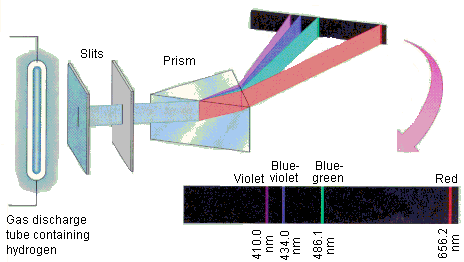These narrow bands have the characteristic wavelengths and colors shown in the table below.

 Wavelength Color 656.2 red 486.1 blue-green 434.0 blue-violet 410.1 violet

Four more series of lines were discovered in the emission spectrum of hydrogen by searching the infrared spectrum at longer wave-lengths and the ultraviolet spectrum at shorter wavelengths. Each of these lines fits the same general equation, where n1 and n2 are integers and RH is 1.09678 x 10-2 nm-1.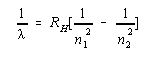Explanation of the Emission Spectrum

Max Planck presented a theoretical explanation of the spectrum of radiation emitted by an object that glows when heated. He argued that the walls of a glowing solid could be imagined to contain a series of resonators that oscillated at different frequencies. These resonators gain energy in the form of heat from the walls of the object and lose energy in the form of electromagnetic radiation. The energy of these resonators at any moment is proportional to the frequency with which they oscillate.

To fit the observed spectrum, Planck had to assume that the energy of these oscillators could take on only a limited number of values. In other words, the spectrum of energies for these oscillators was no longer continuous. Because the number of values of the energy of these oscillators is limited, they are theoretically "countable." The energy of the oscillators in this system is therefore said to be quantized. Planck introduced the notion of quantization to explain how light was emitted.

Albert Einstein extended Planck's work to the light that had been emitted. At a time when everyone agreed that light was a wave (and therefore continuous), Einstein suggested that it behaved as if it was a stream of small bundles, or packets, of energy. In other words, light was also quantized. Einstein's model was based on two assumptions. First, he assumed that light was composed of photons, which are small, discrete bundles of energy. Second, he assumed that the energy of a photon is proportional to its frequency.

E = hv

In this equation, h is a constant known as Planck's constant, which is equal to 6.626 x 10-34 J-s.

Example: Let's calculate the energy of a single photon of red light with a wavelength of 700.0 nm and the energy of a mole of these photons.

Red light with a wavelength of 700.0 nm has a frequency of 4.283 x 1014 s-1. Substituting this frequency into the Planck-Einstein equation gives the following result.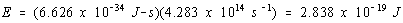A single photon of red light carries an insignificant amount of energy. But a mole of these photons carries about 171,000 joules of energy, or 171 kJ/mol.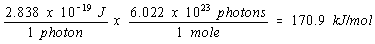Absorption of a mole of photons of red light would therefore provide enough energy to raise the temperature of a liter of water by more than 40oC.

The fact that hydrogen atoms emit or absorb radiation at a limited number of frequencies implies that these atoms can only absorb radiation with certain energies. This suggests that there are only a limited number of energy levels within the hydrogen atom. These energy levels are countable. The energy levels of the hydrogen atom are quantized.

 Learning Activity

 Practice Problem 5:Calculate the energy of a single photon of red light with a wavelength of 700.0 nm and the energy of a mole of these photons. Click here to check your answer to Practice Problem 5 Click here to see a solution to Practice Problem 5The Bohr Model of the Atom

Niels Bohr proposed a model for the hydrogen atom that explained the spectrum of the hydrogen atom. The Bohr model was based on the following assumptions.

• The electron in a hydrogen atom travels around the nucleus in a circular orbit.
• The energy of the electron in an orbit is proportional to its distance from the nucleus. The further the electron is from the nucleus, the more energy it has.
• Only a limited number of orbits with certain energies are allowed. In other words, the orbits are quantized.
• The only orbits that are allowed are those for which the angular momentum of the electron is an integral multiple of Planck's constant divided by 2p.
• Light is absorbed when an electron jumps to a higher energy orbit and emitted when an electron falls into a lower energy orbit.
• The energy of the light emitted or absorbed is exactly equal to the difference between the energies of the orbits.

Some of the key elements of this hypothesis are illustrated in the figure below.Three points deserve particular attention. First, Bohr recognized that his first assumption violates the principles of classical mechanics. But he knew that it was impossible to explain the spectrum of the hydrogen atom within the limits of classical physics. He was therefore willing to assume that one or more of the principles from classical physics might not be valid on the atomic scale.

Second, he assumed there are only a limited number of orbits in which the electron can reside. He based this assumption on the fact that there are only a limited number of lines in the spectrum of the hydrogen atom and his belief that these lines were the result of light being emitted or absorbed as an electron moved from one orbit to another in the atom.

Finally, Bohr restricted the number of orbits on the hydrogen atom by limiting the allowed values of the angular momentum of the electron. Any object moving along a straight line has a momentum equal to the product of its mass (m) times the velocity (v) with which it moves. An object moving in a circular orbit has an angular momentum equal to its mass (m) times the velocity (v) times the radius of the orbit (r). Bohr assumed that the angular momentum of the electron can take on only certain values, equal to an integer times Planck's constant divided by 2p.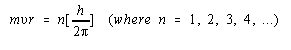Bohr then used classical physics to show that the energy of an electron in any one of these orbits is inversely proportional to the square of the integer n.

The difference between the energies of any two orbits is therefore given by the following equation.In this equation, n1 and n2 are both integers and RH is the proportionality constant known as the Rydberg constant.

Planck's equation states that the energy of a photon is proportional to its frequency.

E = hv

Substituting the relationship between the frequency, wavelength, and the speed of light into this equation suggests that the energy of a photon is inversely proportional to its wavelength. The inverse of the wavelength of electromagnetic radiation is therefore directly proportional to the energy of this radiation.

By properly defining the units of the constant, RH, Bohr was able to show that the wavelengths of the light given off or absorbed by a hydrogen atom should be given by the following equation.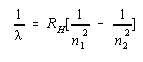Thus, once he introduced his basic assumptions, Bohr was able to derive an equation that matched the relationship obtained from the analysis of the spectrum of the hydrogen atom.

 Practice Problem 6:Calculate the wavelength of the light given off by a hydrogen atom when an electron falls from the n = 4 to the n = 2 orbit in the Bohr model. Click here to check your answer to Practice Problem 6 Click here to see a solution to Practice Problem 6

According to the Bohr model, the wavelength of the light emitted by a hydrogen atom when the electron falls from a high energy (n = 4) orbit into a lower energy (n = 2) orbit.

Substituting the appropriate values of RH, n1, and n2 into the equation shown above gives the following result.Solving for the wavelength of this light gives a value of 486.3 nm, which agrees with the experimental value of 486.1 nm for the blue line in the visible spectrum of the hydrogen atom.Wave-Particle Duality

The theory of wave-particle duality developed by Louis-Victor de Broglie eventually explained why the Bohr model was successful with atoms or ions that contained one electron. It also provided a basis for understanding why this model failed for more complex systems. Light acts as both a particle and a wave. In many ways light acts as a wave, with a characteristic frequency, wavelength, and amplitude. Light carries energy as if it contains discrete photons or packets of energy.

When an object behaves as a particle in motion, it has an energy proportional to its mass (m) and the speed with which it moves through space (s).

E = ms2

When it behaves as a wave, however, it has an energy that is proportional to its frequency: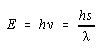By simultaneously assuming that an object can be both a particle and a wave, de Broglie set up the following equation.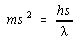By rearranging this equation, he derived a relationship between one of the wave-like properties of matter and one of its properties as a particle.

As noted in the previous section, the product of the mass of an object times the speed with which it moves is the momentum (p) of the particle. Thus, the de Broglie equation suggests that the wavelength (l) of any object in motion is inversely proportional to its momentum.De Broglie concluded that most particles are too heavy to observe their wave properties. When the mass of an object is very small, however, the wave properties can be detected experimentally. De Broglie predicted that the mass of an electron was small enough to exhibit the properties of both particles and waves. In 1927 this prediction was confirmed when the diffraction of electrons was observed experimentally by C. J. Davisson.

De Broglie applied his theory of wave-particle duality to the Bohr model to explain why only certain orbits are allowed for the electron. He argued that only certain orbits allow the electron to satisfy both its particle and wave properties at the same time because only certain orbits have a circumference that is an integral multiple of the wavelength of the electron, as shown in the figure below.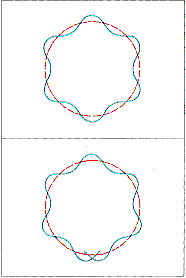The Bohr Model vs. Reality

At first glance, the Bohr model looks like a two-dimensional model of the atom because it restricts the motion of the electron to a circular orbit in a two-dimensional plane. In reality the Bohr model is a one-dimensional model, because a circle can be defined by specifying only one dimension: its radius, r. As a result, only one coordinate (n) is needed to describe the orbits in the Bohr model.

Unfortunately, electrons aren't particles that can be restricted to a one-dimensional circular orbit. They act to some extent as waves and therefore exist in three-dimensional space. The Bohr model works for one-electron atoms or ions only because certain factors present in more complex atoms are not present in these atoms or ions. To construct a model that describes the distribution of electrons in atoms that contain more than one electron we have to allow the electrons to occupy three-dimensional space. We therefore need a model that uses three coordinates to describe the distribution of electrons in these atoms.Wave Functions and Orbitals

We still talk about the Bohr model of the atom even if the only thing this model can do is explain the spectrum of the hydrogen atom because it was the last model of the atom for which a simple physical picture can be constructed. It is easy to imagine an atom that consists of solid electrons revolving around the nucleus in circular orbits.

Erwin Schrödinger combined the equations for the behavior of waves with the de Broglie equation to generate a mathematical model for the distribution of electrons in an atom. The advantage of this model is that it consists of mathematical equations known as wave functions that satisfy the requirements placed on the behavior of electrons. The disadvantage is that it is difficult to imagine a physical model of electrons as waves.

The Schrödinger model assumes that the electron is a wave and tries to describe the regions in space, or orbitals, where electrons are most likely to be found. Instead of trying to tell us where the electron is at any time, the Schrödinger model describes the probability that an electron can be found in a given region of space at a given time. This model no longer tells us where the electron is; it only tells us where it might be.